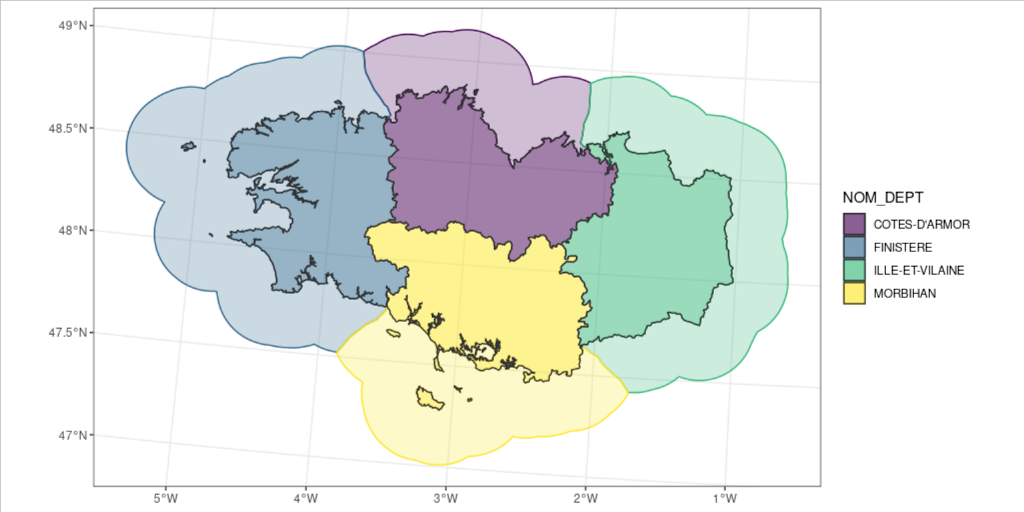# Buffer areas for nearest neighbours or national marine areas delineation

A few weeks ago, Christina Buelow asked on Twitter how she could create the polygons of coastal waters using the land polygons, and making sure there is no overlap in buffer areas. This would make it possible to know which land area is closest to any place in the sea. Let’s explore what solution emerged from the discussions.

## TL;DR

``````# Define where to save the dataset
extraWD <- tempdir()
# Get some data available to anyone
if (!file.exists(file.path(extraWD, "departement.zip"))) {
githubURL <- "https://github.com/statnmap/blog_tips/raw/master/2018-07-14-introduction-to-mapping-with-sf-and-co/data/departement.zip"
unzip(file.path(extraWD, "departement.zip"), exdir = extraWD)
}``````
• Reduce the dataset to a small region
``````library(sf)
# remotes::install_github("statnmap/cartomisc")
library(cartomisc)
library(dplyr)
library(ggplot2)

departements_l93 <- read_sf(dsn = extraWD, layer = "DEPARTEMENT")

# Reduce dataset
bretagne_l93 <- departements_l93 %>%
filter(NOM_REG == "BRETAGNE")``````
• Calculate the regional buffer area using `regional_seas()`
``````bretagne_regional_2km_l93 <- regional_seas(
x = bretagne_l93,
group = "NOM_DEPT",
dist = units::set_units(30, km), # buffer distance
density = units::set_units(0.5, 1/km) # density of points (the higher, the more precise the region attribution)
)``````
• Plot the data
``````ggplot() +
geom_sf(data = bretagne_regional_2km_l93,
aes(colour = NOM_DEPT, fill = NOM_DEPT),
alpha = 0.25) +
geom_sf(data = bretagne_l93,
aes(fill = NOM_DEPT),
colour = "grey20",
alpha = 0.5) +
scale_fill_viridis_d() +
scale_color_viridis_d() +
theme_bw()``````If you want to know the long story, you can continue reading this blog post, otherwise, you have everything you need to start on your own data !

## Create buffer area around adjacent polygons

As always when trying to figure out a solution to a specific problem, let’s start with a reproducible example, commonly called “reprex” among R users. This means, we create the minimal code that allows anyone to execute the code, face the same problem and show everything we tested. In the present case, I couldn’t find enough time to reach a solution during the discussions, so I prepared a gist showing what I tried, what point I reached, and more importantly what I plan to achieve.

``````library(sf)
library(ggplot2)
library(dplyr)

my_blue <- "#1e73be"``````

``````# Define where to save the dataset
extraWD <- "."
# Get some data available to anyone
if (!file.exists(file.path(extraWD, "departement.zip"))) {
githubURL <- "https://github.com/statnmap/blog_tips/raw/master/2018-07-14-introduction-to-mapping-with-sf-and-co/data/departement.zip"
unzip(file.path(extraWD, "departement.zip"), exdir = extraWD)
}``````

I reduce the dataset to better see what I am doing. Here, I filter the Brittany area.
As you can see, there are 4 departments in this region, thus 4 spatial features.

``````departements_l93 <- read_sf(dsn = extraWD, layer = "DEPARTEMENT")

# Reduce dataset
bretagne_l93 <- departements_l93 %>%
filter(NOM_REG == "BRETAGNE")

bretagne_l93``````
``````## Simple feature collection with 4 features and 11 fields
## Geometry type: MULTIPOLYGON
## Dimension:     XY
## Bounding box:  xmin: 99217.1 ymin: 6704195 xmax: 400572.3 ymax: 6881964
## Projected CRS: RGF93_Lambert_93
## # A tibble: 4 x 12
##   ID_GEOFLA CODE_DEPT NOM_DEPT CODE_CHF NOM_CHF X_CHF_LIEU Y_CHF_LIEU X_CENTROID
## * <chr>     <chr>     <chr>    <chr>    <chr>        <int>      <int>      <int>
## 1 DEPARTEM… 35        ILLE-ET… 238      RENNES      351870    6789646     326237
## 2 DEPARTEM… 22        COTES-D… 278      SAINT-…     274947    6839206     260216
## 3 DEPARTEM… 29        FINISTE… 232      QUIMPER     171326    6789950     115669
## 4 DEPARTEM… 56        MORBIHAN 260      VANNES      267889    6744074     256790
## # … with 4 more variables: Y_CENTROID <int>, CODE_REG <chr>, NOM_REG <chr>,
## #   geometry <MULTIPOLYGON [m]>``````

Now, I can plot the output of buffer areas around separate polygons.

``````# Create buffer
bretagne_buffer_l93 <- bretagne_l93 %>%
st_buffer(
dist =
units::set_units(10, km)
)

ggplot() +
geom_sf(data = bretagne_buffer_l93, fill = my_blue, alpha = 0.2, colour = my_blue) +
geom_sf(data = bretagne_l93, fill = NA) +
theme_bw()``````You can see there are overlapping areas.
This is where Voronoï polygons come to help us. From here, I will take some ideas that Christina gathered during her Twitter discussions. Her complete code for coastal buffers is here.

``````# Create a merged region entity
bretagne_union_l93 <- bretagne_l93 %>%
summarise()

ggplot() +
geom_sf(data = bretagne_union_l93, colour = my_blue, fill = NA)``````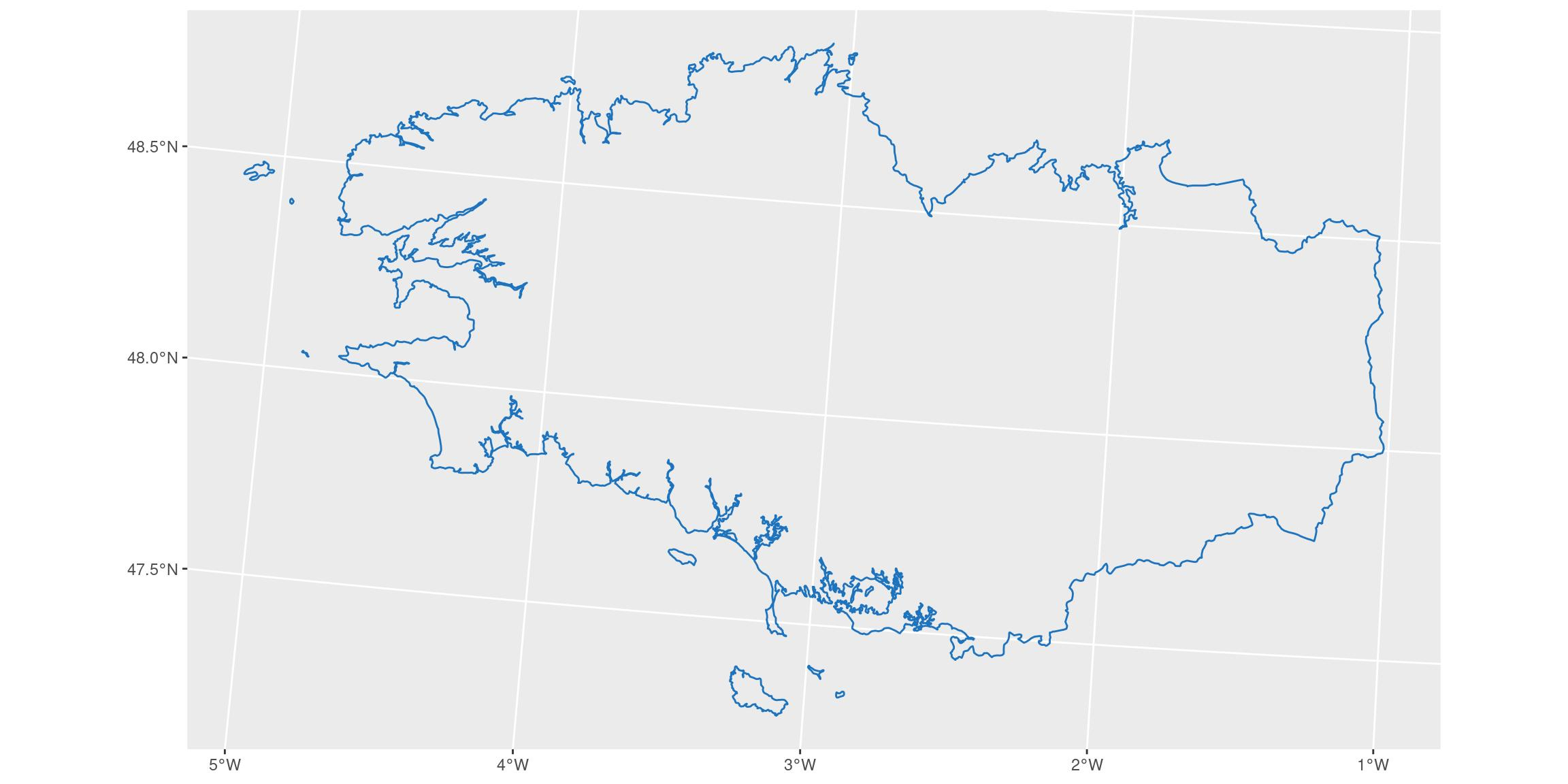``````# Create a doughnut for regional seas areas, 30km off coasts
bretagne_seas_l93 <- bretagne_union_l93 %>%
st_buffer(
dist = units::set_units(30, km)
) %>%
st_cast()

ggplot() +
geom_sf(data = bretagne_seas_l93, colour = my_blue, fill = NA)``````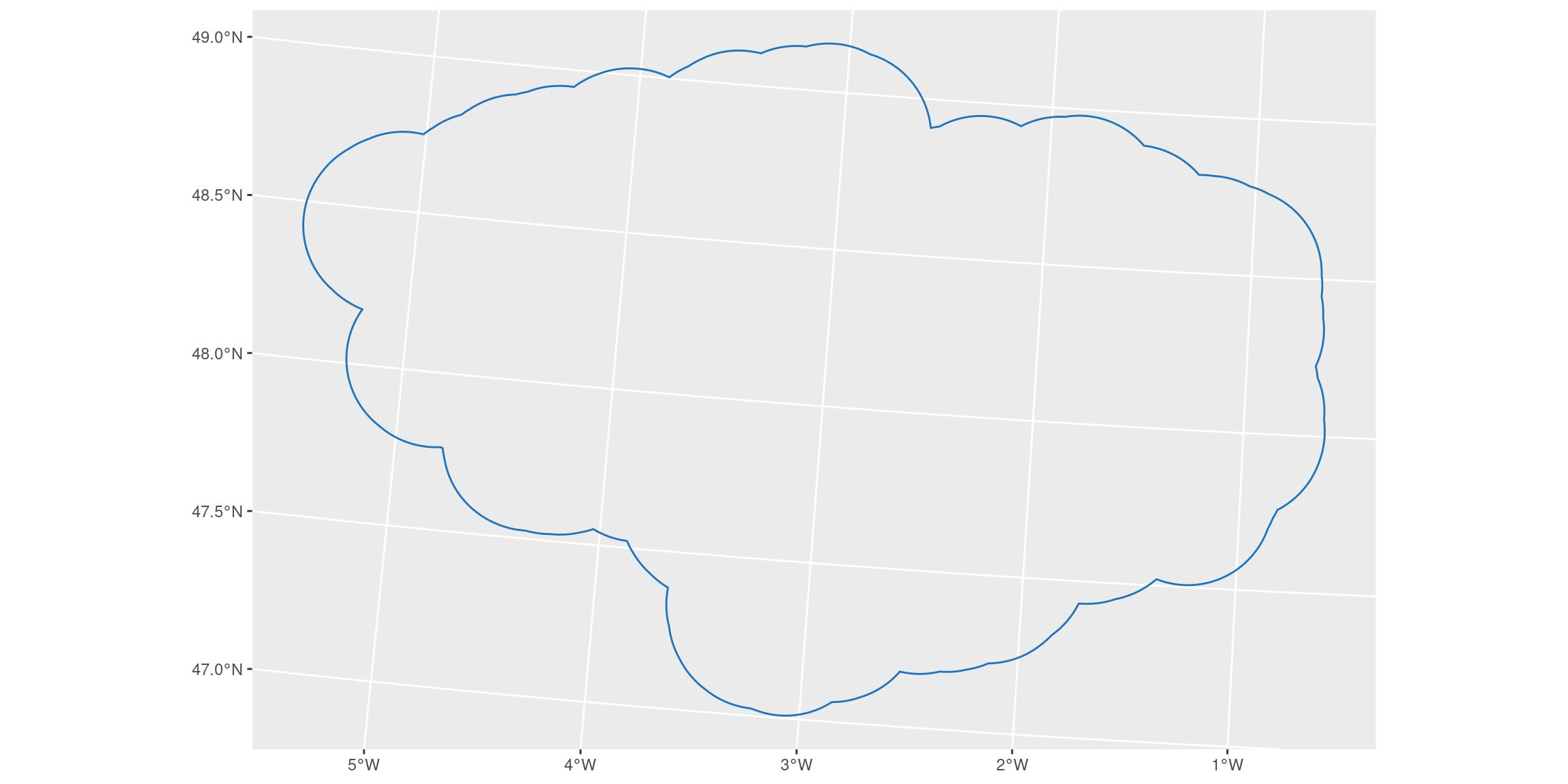``````# Remove inside terrestrial parts
bretagne_donut_l93 <- bretagne_seas_l93 %>%
st_difference(bretagne_union_l93) %>%
st_cast()

ggplot() +
geom_sf(data = bretagne_donut_l93, colour = my_blue, fill = my_blue, alpha = 0.2)``````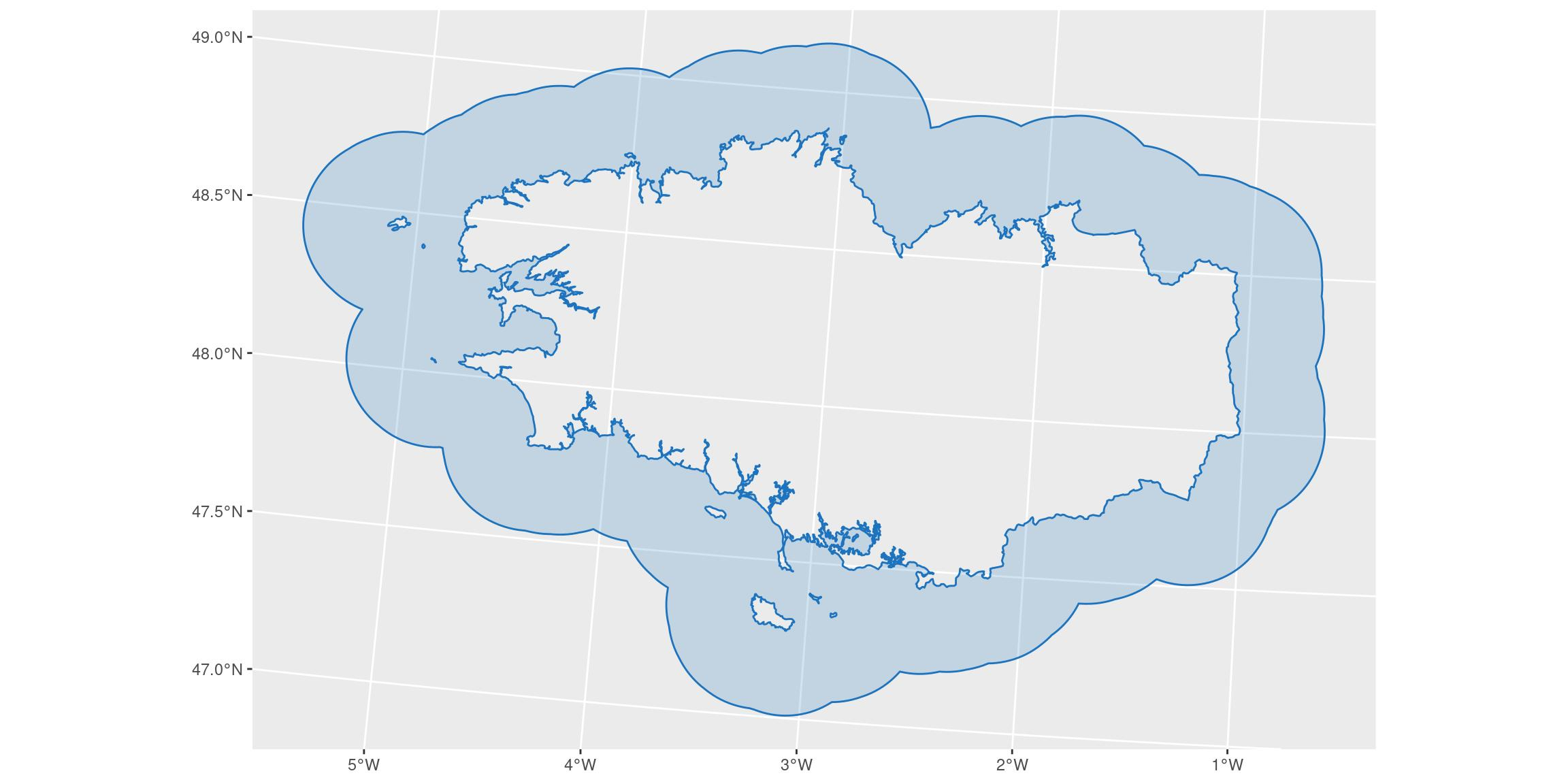## Let’s divide this area in multiples polygons

We use the coastlines as a basis.

``````# density <- units::set_units(0.1, 1/km)

# First merge everything and transform as lines
bretagne_points_l93 <- bretagne_union_l93 %>%
# transform polygons as multi-lines
st_cast("MULTILINESTRING") %>%
# transform multi-lines as separate unique lines
st_cast("LINESTRING") %>%
# transform as points, 0.1 per km (= 1 every 10 km)
# Choose density according to precision needed
st_line_sample(density = units::set_units(0.1, 1/km)) %>%
st_sf() #%>%
# remove empty geometry (small islands where sample is not possible with this density)
# filter(!st_is_empty(geometry))

ggplot() +
geom_sf(data = bretagne_points_l93, colour = my_blue, fill = NA)``````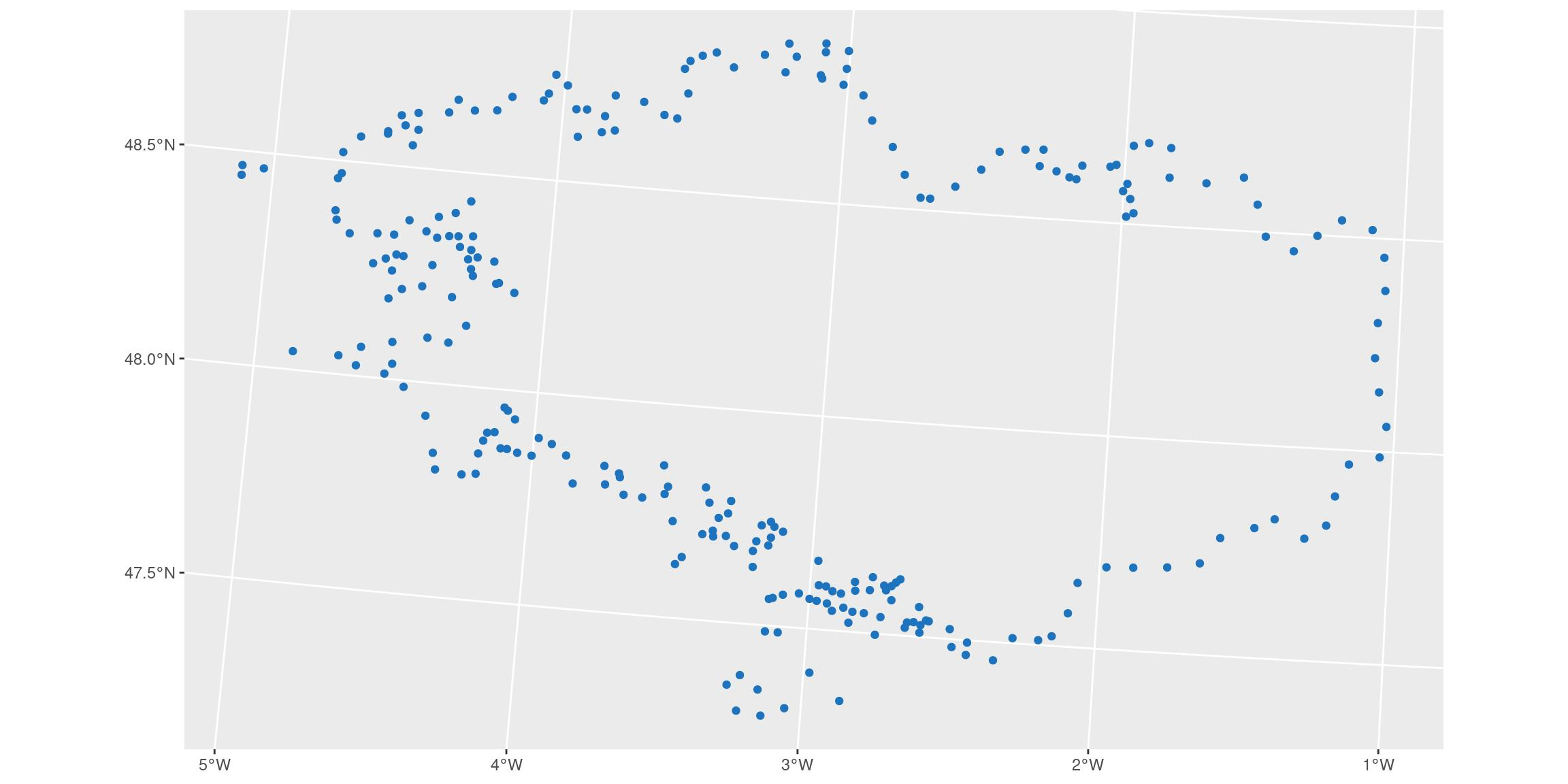``````# Create voronoi polygons around points
bretagne_voronoi_l93 <- bretagne_points_l93 %>%
st_combine() %>%
st_voronoi() %>%
st_cast()

ggplot() +
geom_sf(data = bretagne_voronoi_l93, colour = my_blue, fill = NA)``````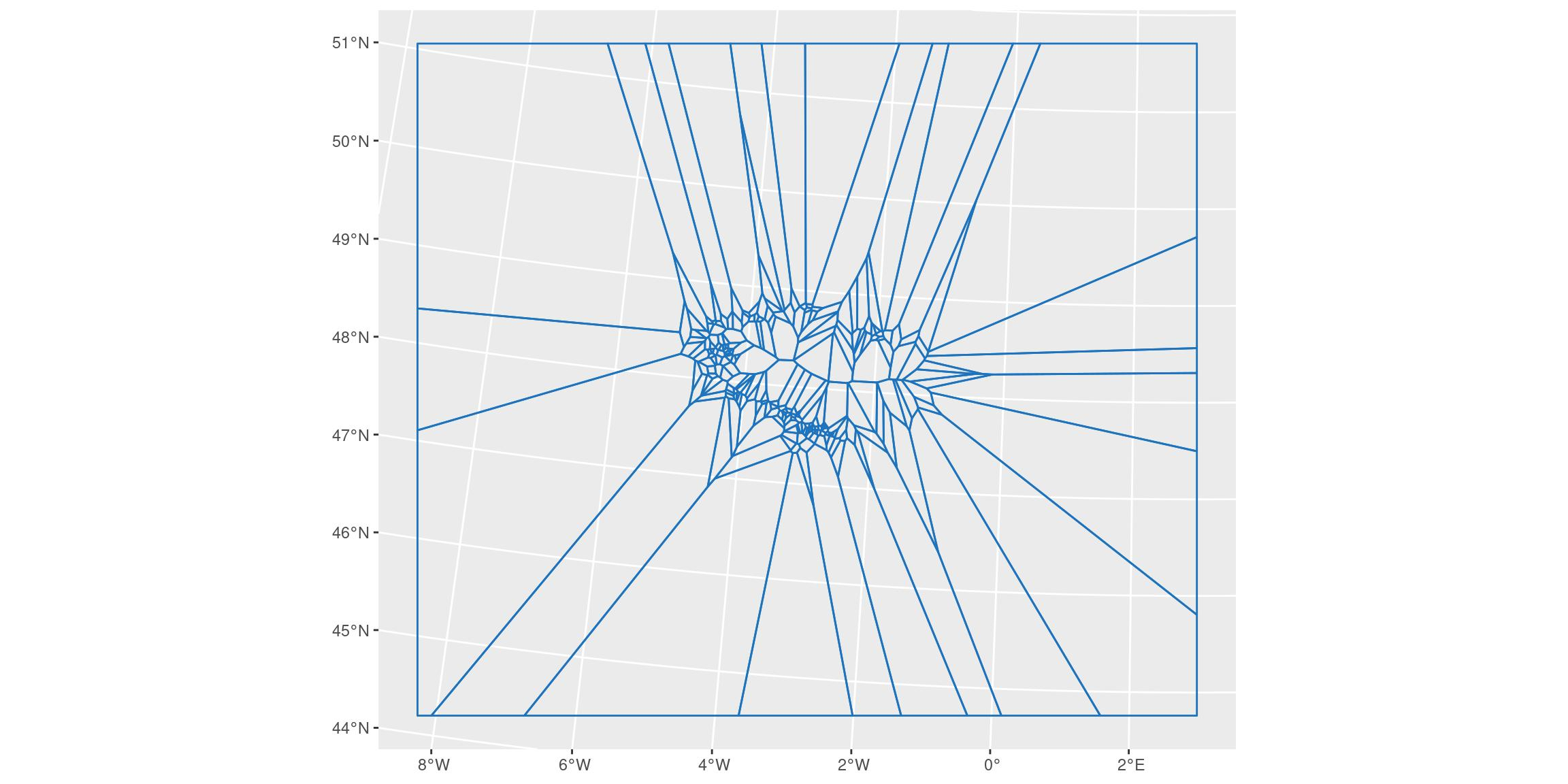## Join voronoi and donut polygon

``````bretagne_voronoi_donut_l93 <- bretagne_voronoi_l93 %>%
st_intersection(bretagne_donut_l93) %>%
st_cast() %>%
st_sf()

ggplot() +
geom_sf(data = bretagne_voronoi_donut_l93, colour = my_blue, fill = NA)``````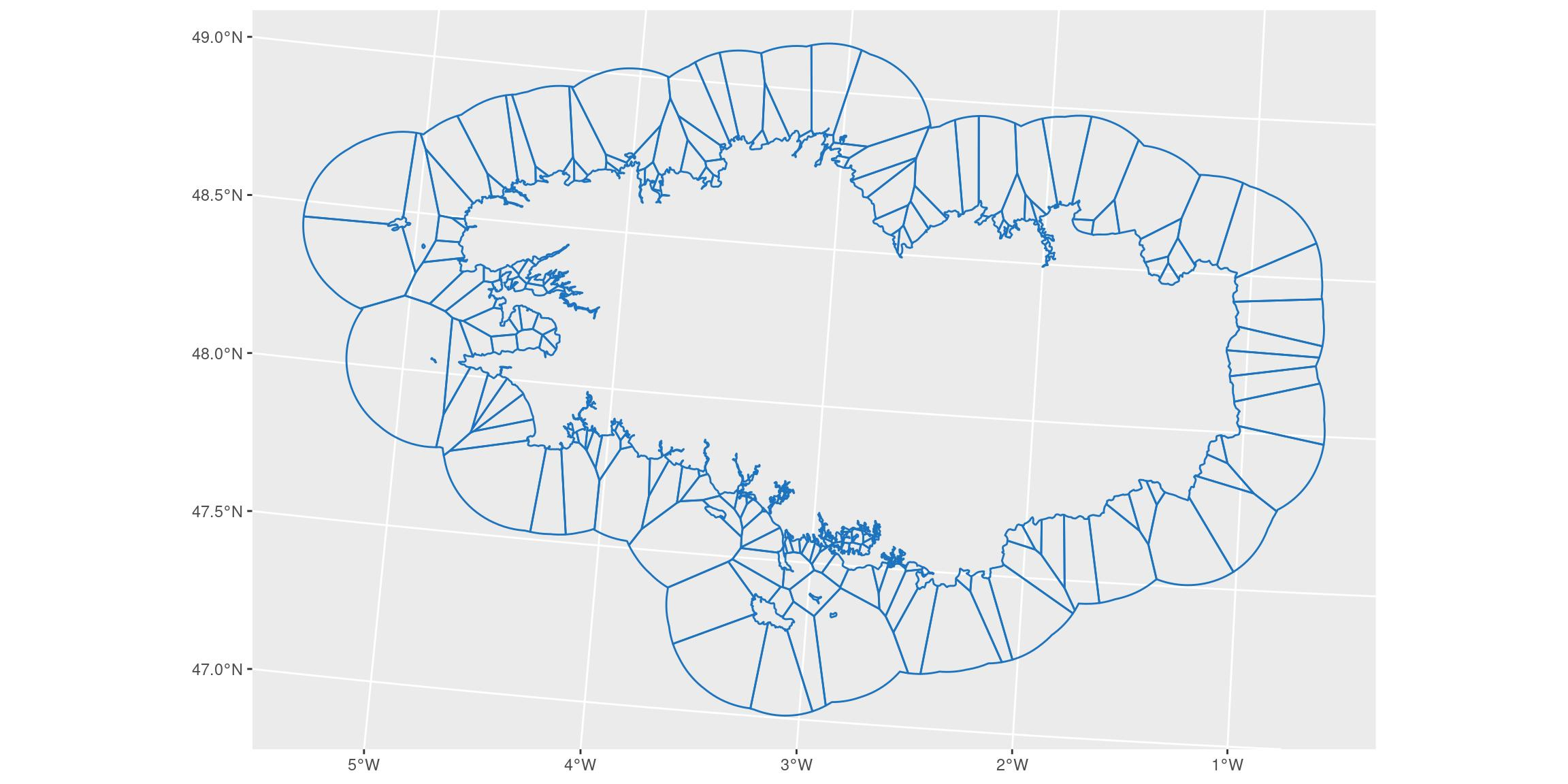# Join voronoi-donut polygons to original dataset to intersect with regions

We realise sort of a `left_join` based on spatial attributes using `st_join()`. This will keep the original geometry of our voronoi-donut polygons, while retrieving variables of joined terrestrial dataset when there is intersection.

``````# Equivalent to left_join
bretagne_vd_region_l93 <- bretagne_voronoi_donut_l93 %>%
st_join(bretagne_l93)

ggplot() +
geom_sf(data = bretagne_vd_region_l93,
aes(colour = NOM_DEPT, fill = NOM_DEPT),
alpha = 0.25)````````````# Interactive view
mapview::mapview(bretagne_vd_region_l93, zcol = "NOM_DEPT")``````

If we zoom on the map using `mapview()`, you can see that polygons between two regions sees a double attribution. This is because voronoi intersect with both regions. If you want to reduce the size of the overlapping polygons, you can increase the density of points used to create the voronoi polygons in `st_line_sample()`. However, we will always have the double attribution. Remember that `st_join()` works like `left_join()`. If there are duplicate keys, entities are doubled.
To avoid this, we will have to choose between one region or the other. First, we give a number to the voronoi polygons before joining to be able to identify those that are doubled. Then, we will choose to keep only the first of them in the list. Note that `summarise_all` does not exists for {sf} objects. I cannot use `group_by(id_v) + summarise_all(first)` as for classical datasets, neither the new `across()` function. I use `distinct()` with parameter `keep_all = TRUE` so that it keeps all variables with the first entity for each voronoi polygon.

``````bretagne_vd_region_distinct_l93 <- bretagne_voronoi_donut_l93 %>%
mutate(id_v = 1:n()) %>%
st_join(bretagne_l93) %>%
# group_by(id_v) %>%
# summarise(across(.cols = everything(), .fns = first)) # Not on {sf}
# summarise_all(.funs = first) # Not on {sf}
distinct(id_v, .keep_all = TRUE)

ggplot() +
geom_sf(data = bretagne_vd_region_distinct_l93,
aes(colour = NOM_DEPT, fill = NOM_DEPT),
alpha = 0.25)``````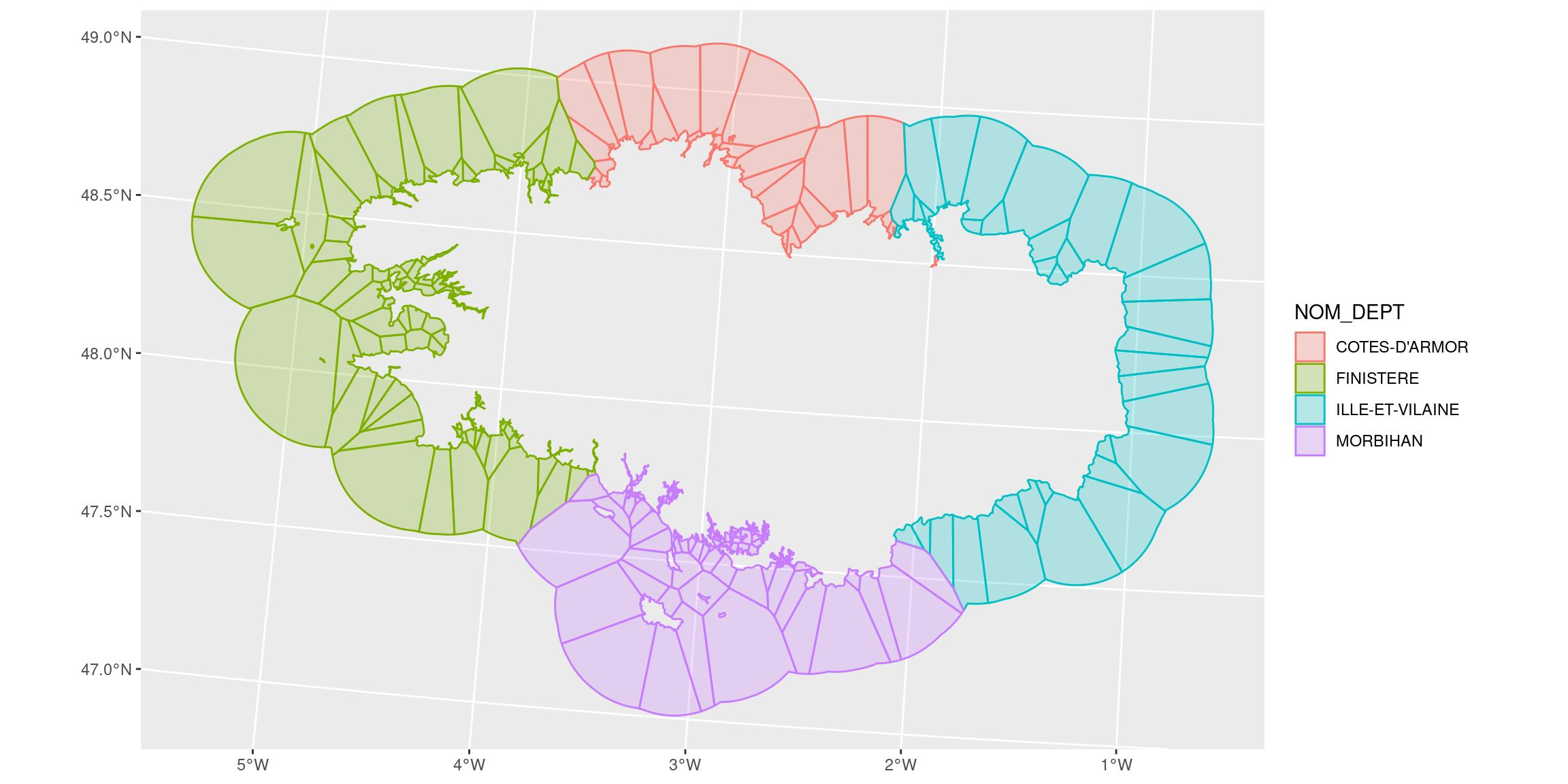``````# Interactive view
mapview::mapview(bretagne_vd_region_distinct_l93, zcol = "NOM_DEPT")``````